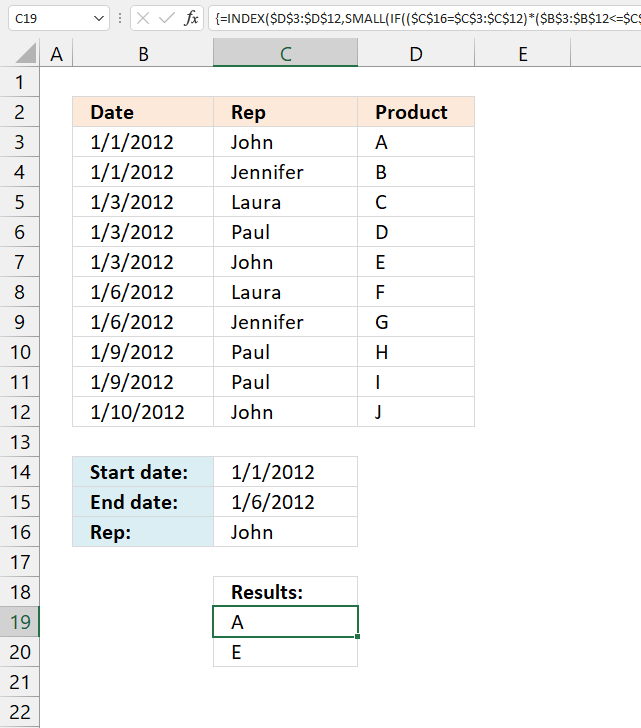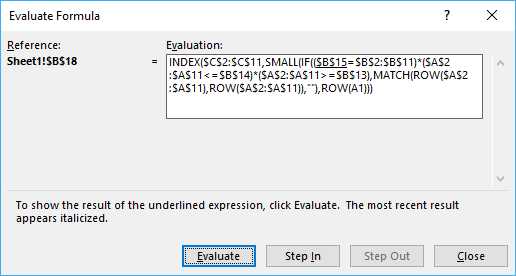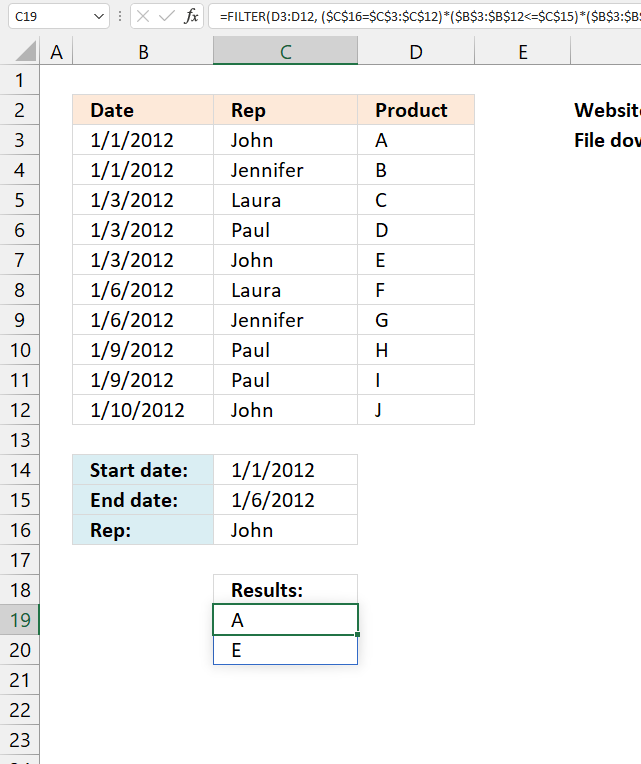Author: Oscar Cronquist Article last updated on August 25, 2021This article demonstrates a formula that extracts values from a column based on a date range criteria and another condition.

For example, column B contains dates. Column C contains names, column D contains products. The formula in cell C19 extracts products based on the date range specified in cells C14:C15 and a condition in cell C16.

## 1. Lookup a date range and a condition - return multiple valuesJason C asks:I have a set of data, like the one you used in the original example that also has a column for the date of the transaction. I would like my Index-type formula to search for both the main item (the rep's name) and also if the date of the transaction falls in the date range).

Start Date: 11/26/2012
End Date: 11/30/2012 (both entered by the user)
Rep: John

Then the results, in each row/column of the 'result' section (INDEX formula results) would show results for John that occurred from 11/26 to 11/30 (including both dates).

Thanks for any help with the formula for that.

Jason

The image above demonstrates a formula in cell range B18:B19 that extracts values from column C if the corresponding value in column B is between two given dates and the corresponding value in column A matches a specified value.

The following formula is for earlier Excel versions, array formula in cell B18:

=INDEX(\$C\$2:\$C\$11, SMALL(IF((\$B\$15=\$B\$2:\$B\$11)*(\$A\$2:\$A\$11<=\$B\$14)*(\$A\$2:\$A\$11>=\$B\$13), MATCH(ROW(\$A\$2:\$A\$11), ROW(\$A\$2:\$A\$11)), ""), ROW(A1)))

### 1.1 How to create an array formula

1. Copy above array formula.
2. Double press with left mouse button on cell E2, the prompt appears.
3. Paste array formula.
4. Press and hold Ctrl + Shift simultaneously.
5. Press Enter once.
6. Release all keys.

A beginning and ending curly bracket appears like this {=array_formula}. Don't enter these characters yourself, they show up automatically.

### 1.2 How to copy array formula

1. Select cell E2
2. Copy cell (Ctrl + c)
3. Select cell E3:E20
4. Paste (Ctrl + v)

### 1.3 Explaining array formula in cell B18

You can easily follow along, select cell B18. Go to tab "Formulas" and press with left mouse button on the "Evaluate formula" button.Press with left mouse button on "Evaluate" button, shown in above picture, to move to next step.

#### Step 1 - Compare value in cell B15 to cell range \$B\$2:\$B\$11

\$B\$15=\$B\$2:\$B\$11

becomes

"John"={"John";"Jennifer";"Laura";"Paul";"John";"Laura";"Jennifer";"Paul";"Paul";"John"}

and returns

{TRUE;FALSE;FALSE;FALSE;TRUE;FALSE;FALSE;FALSE;FALSE;TRUE}

#### Step 2 - Compare start and end date to date column

(\$A\$2:\$A\$11<=\$B\$14)*(\$A\$2:\$A\$11>=\$B\$13)

becomes

({TRUE; TRUE; TRUE; TRUE; TRUE; TRUE; TRUE; FALSE; FALSE; FALSE})*({TRUE; TRUE; TRUE; TRUE; TRUE; TRUE; TRUE; TRUE; TRUE; TRUE})

and returns

{1;1;1;1;1;1;1;0;0;0}

#### Step 3 - If a record is a match return it´s row number

IF((\$B\$15=\$B\$2:\$B\$11)*(\$A\$2:\$A\$11<=\$B\$14)*(\$A\$2:\$A\$11>=\$B\$13),MATCH(ROW(\$A\$2:\$A\$11),ROW(\$A\$2:\$A\$11))

becomes

IF({TRUE; FALSE; FALSE; FALSE; TRUE; FALSE; FALSE; FALSE; FALSE; TRUE}*{1;1;1;1;1;1;1;0;0;0},MATCH(ROW(\$A\$2:\$A\$11),ROW(\$A\$2:\$A\$11))

becomes

IF({1;0;0;0;1;0;0;0;0;0},{1; 2; 3; 4; 5; 6; 7; 8; 9; 10})

and returns

{1;FALSE;FALSE;FALSE;5;FALSE;FALSE;FALSE;FALSE;FALSE}

Recommended articles

How to use the IF function
Checks if a logical expression is met. Returns a specific value if TRUE and another specific value if FALSE.

#### Step 4 - Return the k-th smallest value

SMALL(IF((\$B\$15=\$B\$2:\$B\$11)*(\$A\$2:\$A\$11<=\$B\$14)*(\$A\$2:\$A\$11>=\$B\$13),MATCH(ROW(\$A\$2:\$A\$11),ROW(\$A\$2:\$A\$11)),""),ROW(A1))

becomes

SMALL({1; FALSE; FALSE; FALSE; 5; FALSE; FALSE; FALSE; FALSE; FALSE},ROW(A1))

becomes

SMALL({1; FALSE; FALSE; FALSE; 5; FALSE; FALSE; FALSE; FALSE; FALSE},1)

and returns 1.

Recommended articles

How to use the SMALL function
The SMALL function lets you extract a number in a cell range based on how small it is compared to the other numbers in the group.

#### Step 5 - Return the value of a cell at the intersection of a particular row and column

INDEX(\$C\$2:\$C\$11,SMALL(IF((\$B\$15=\$B\$2:\$B\$11)*(\$A\$2:\$A\$11<=\$B\$14)*(\$A\$2:\$A\$11>=\$B\$13),MATCH(ROW(\$A\$2:\$A\$11),ROW(\$A\$2:\$A\$11)),""),ROW(A1)))

becomes

INDEX(\$C\$2:\$C\$11,1)

becomes

INDEX({"A"; "B"; "C"; "D"; "E"; "F"; "G"; "H"; "I"; "J"},1)

and returns A in cell B18.

Recommended articles

How to use the INDEX function
Gets a value in a specific cell range based on a row and column number.

## 2. Lookup a date range and a condition return multiple values - Excel 365Update 2020-12-17, the new FILTER function is available for Excel 365 users. The regular formula in cell B18:

=FILTER(D3:D12, (\$C\$16=\$C\$3:\$C\$12)*(\$B\$3:\$B\$12<=\$C\$15)*(\$B\$3:\$B\$12>=\$C\$14))

The FILTER function is a dynamic array formula meaning it returns an array of values to cell B18 and cells below automatically.

### 2.1 Explaining formula

#### Step 1 - Check which dates are larger or equal to the start date condition

The greater than and equal sign combined creates a logical test and the output is either True or False.

\$B\$3:\$B\$12>=\$C\$14

becomes

{40909; 40909; 40911; 40911; 40911; 40914; 40914; 40917; 40917; 40918}>=40909

and returns

{TRUE; TRUE; TRUE; TRUE; TRUE; TRUE; TRUE; TRUE; TRUE; TRUE}.

#### Step 2 - Check which dates are smaller or equal to end date condition

\$B\$3:\$B\$12<=\$C\$15

becomes

{40909; 40909; 40911; 40911; 40911; 40914; 40914; 40917; 40917; 40918}<=40914

and returns

{TRUE; TRUE; TRUE; TRUE; TRUE; TRUE; TRUE; FALSE; FALSE; FALSE}

#### Step 3 - Condition

\$C\$16=\$C\$3:\$C\$12

becomes

"John"={"John"; "Jennifer"; "Laura"; "Paul"; "John"; "Laura"; "Jennifer"; "Paul"; "Paul"; "John"}

and returns

{TRUE; FALSE; FALSE; FALSE; TRUE; FALSE; FALSE; FALSE; FALSE; TRUE}

#### Step 4 - Multiply arrays - AND logic

The asterisk character multiplies the logical tests, this applies AND logic meaning:

TRUE * TRUE = TRUE
FALSE * TRUE = FALSE
TRUE * FALSE = FALSE
FALSE * FALSE = FALSE

(\$C\$16=\$C\$3:\$C\$12)*(\$B\$3:\$B\$12<=\$C\$15)*(\$B\$3:\$B\$12>=\$C\$14)

becomes

{TRUE; TRUE; TRUE; TRUE; TRUE; TRUE; TRUE; TRUE; TRUE; TRUE} * {TRUE; TRUE; TRUE; TRUE; TRUE; TRUE; TRUE; TRUE; TRUE; TRUE} * {TRUE; FALSE; FALSE; FALSE; TRUE; FALSE; FALSE; FALSE; FALSE; TRUE}

and returns

{1; 0; 0; 0; 1; 0; 0; 0; 0; 0}.

#### Step 5 - Filter values based on criteria

The FILTER function lets you extract values/rows based on a condition or criteria. It is in the Lookup and reference category and is only available to Excel 365 subscribers.

FILTER(D3:D12, (\$C\$16=\$C\$3:\$C\$12)*(\$B\$3:\$B\$12<=\$C\$15)*(\$B\$3:\$B\$12>=\$C\$14))

becomes

FILTER({"A"; "B"; "C"; "D"; "E"; "F"; "G"; "H"; "I"; "J"}, {1; 0; 0; 0; 1; 0; 0; 0; 0; 0})

and returns {"A";"E"}.